# Abaqus Plug-In (desicos.abaqus)¶

The desicos.abaqus module includes the DESICOS plug-in for Abaqus whose functionalities can be used in two main ways, using the Graphic User Interface (GUI) or using the Python API.

## ConeCyl (desicos.abaqus.conecyl)¶

### Cone/Cylinder Model¶

Figure 1 provides a schematic view of the typical model created using this module. Two coordinate systems are defined: one rectangular with axes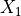,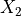,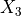and a cylindrical with axes,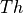,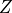.

The complexity of the actual model created in Abaqus goes beyond the simplification above

### Boundary Conditions¶

Based on the coordinate systems shown in Figure 1 the following boundary condition parameters can be controlled:

• constraint for radial and circumferential displacement (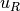and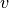) at the bottom and top edges

• simply supported or clamped bottom and top edges, consisting in the rotational constraint along the meridional coordinate, called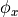.

• use of resin rings as described in the next section

• the use of distributed or concentrated load at the top edge will be automatically determined depending on the attributes of the current ConeCyl object

• application of shims at the top edge as detailed in ImpConf.add_shim_top_edge(), following this example:

from desicos.abaqus.conecyl import ConeCyl

cc = ConeCyl()
cc.from_DB('castro_2014_c02')

• application of uneven top edges as detailed in UnevenTopEdge.add_measured_u3s(), following this example:

thetadegs = [0.0, 22.5, 45.0, 67.5, 90.0, 112.5, 135.0, 157.5, 180.0,
202.5, 225.0, 247.5, 270.0, 292.5, 315.0, 337.5, 360.0]
u3s = [0.0762, 0.0508, 0.1270, 0.0000, 0.0000, 0.0762, 0.2794, 0.1778,
0.0000, 0.0000, 0.0762, 0.0000, 0.1016, 0.2032, 0.0381, 0.0000,
0.0762]


### Resin Rings¶

When resin rings are used the actual boundary condition will be determined by the parameters defining the resin rings (cf. Figure 2), and therefore no clamped conditions will be applied in the shell edges.

Defining resin rings can be done following the example below, where each attribute is detailed in the ConeCyl class description:

from desicos.abaqus.conecyl import ConeCyl

cc = Conecyl()
cc.from_DB('castro_2014_c02')
cc.resin_E = 2454.5336
cc.resin_nu = 0.3
cc.resin_numel = 3
cc.resin_bot_h = 25.4
cc.resin_top_h = 25.4
cc.resin_bir_w1 = 25.4
cc.resin_bir_w2 = 25.4
cc.resin_bor_w1 = 25.4
cc.resin_bor_w2 = 25.4
cc.resin_tir_w1 = 25.4
cc.resin_tir_w2 = 25.4
cc.resin_tor_w1 = 25.4
cc.resin_tor_w2 = 25.4


### The ConeCyl Class¶

class desicos.abaqus.conecyl.conecyl.ConeCyl[source]

ConeCyl object

Carries all the information necessary to create a finite element model for the analysis of conical and cylindrical structures. The tables below show the attributes grouped by category.

General Attributes

Description

name_DB

str, name of the corresponding desicos.conecylDB.ccs entry

model_name

str, Name of the corresponding model in Abaqus

rename

bool, tells to automatically rename during rebuild()

rebuilt

bool, tells if rebuild() already finished

created_model

bool, tells if the corresponding model was already created in Abaqus

impconf

The corresponding imperfection configuration (see ImpConf)

stringerconf

The corresponding stringer configuration (see StringerConf)

Geometric Attributes

Description

rbot

rtop

H

Height

L

Meridional length (same as H for cylinders)

alphadeg

Cone semi-vertex angle in degrees

Laminate Attributes

Description

stack

list, stacking sequence with angles in degrees

plyt

float, ply thickness that will be used for all plies

plyts

list, ply thicknesses for each ply (overwrites plyt if both are given). If this has a different length than stack, the first thickness will be applied for all plies

laminapropKey

str, name of the lamina properties contained in the database (see conecylDB.laminaprops

laminapropKeys

list a list of strings when different lamina property names are given for each ply (overwrites laminapropKey when given)

laminaprop

tuple, lamina properties given as (E11, E22, nu12, G12, G13, G23)

laminaprops

list a list of tuples when different lamina properties should be used for each ply (overwrites laminaprop, laminapropKey and laminapropKeys, when given)

allowable

tuple, lamina allowables given as (S11t, S11c, S22t, S22c, S12, S13)

allowables

list a list of tuples when different lamina allowables should be used for each ply

Description

displ_controlled

bool, if the axial compression is displacement controlled

pressure_load

float, the pressure load to be applied (a positive value will create a positive pressure, if None, False, 0 no pressure is applied)

pressure_step

int, if pressure should be applied in the first (constant) or second (incremented) step

axial_displ

float, the axial displacement

Note

Applicable if displ_controlled=True

axial_load

float, the axial load

Note

Applicable if displ_controlled=False

axial_step

int, if the axial load should be applied in the first (constant) or second (incremented) step

Nxxtop

str, allows the use of a general equation for the distributed force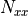at the top edge. The coordinates of the top edge are given in cylindrical coordinates: R, Th, Z; and common functions like cos, sin, tan, acos, asin, atan, pow and constants like pi, e etc can be used. Example:

cc.Nxxtop = "cos(Th)+sin(Th)"


Note

If Nxxtop is not given, the formula: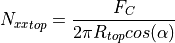will be adopted, where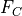is the axial_load attribute

Note

Applicable if displ_controlled=False

Nxxtop_vec

tuple, the direction to apply Nxxtop. Applicable only when Nxxtop is defined. This vector is defined with two points in the cylindrical coordinate system of Figure 1: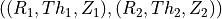If no direction is given Nxxtop will be applied along the shell membrane direction

Note

Applicable if displ_controlled=False

linear_buckling

bool, tells if the current model is for linear buckling analysis. If True the created model will have no imperfection and only an unitary axial load applied at the top edge

Note

The routines automatically determine whether the load should be distributed or applied in a reference point based on the defined boundary conditions

Boundary Conditions

Description

bc_fix_bottom_uR

bool, if the radial displacement should be constrained at the bottom edge (cf. Figure 1)

bc_fix_bottom_v

bool, if the circumferential displacement should be constrained at the bottom edge (cf. Figure 1)

bc_bottom_clamped

bool, if the bottom edge should be clamped

Note

Until version 2.1.3 (inclusive), this setting would not apply if cc.resin_add_BIR or cc.resin_add_BOR

bc_fix_bottom_side_uR

bool, if the radial displacement should be constrained at the inner / outer side faces of the bottom resin rings (when present).

bc_fix_bottom_side_v

bool, if the circumferential displacement should be constrained at the inner / outer side faces of the bottom resin rings (when present).

bc_fix_bottom_side_u3

bool, if the vertical displacement should be should be constrained at the inner / outer side faces of the bottom resin rings (when present).

bc_fix_top_uR

bool, if the radial displacement should be constrained at the top edge (cf. Figure 1)

bc_fix_top_v

bool, if the circumferential displacement should be constrained at the top edge (cf. Figure 1)

bc_top_clamped

bool, if the top edge should be clamped

Note

Until version 2.1.3 (inclusive), this setting would not apply if cc.resin_add_TIR or cc.resin_add_TOR

bc_fix_top_side_uR

bool, if the radial displacement should be constrained at the inner / outer side faces of the top resin rings (when present).

bc_fix_top_side_v

bool, if the circumferential displacement should be constrained at the inner / outer side faces of the top resin rings (when present).

bc_fix_top_side_u3

bool, if the vertical displacement should be should be constrained at the inner / outer side faces of the top resin rings (when present).

Resin Rings

Description (the attributes are illustrated here)

resin_add_BIR

bool, tells if a resin ring should be added to the inner part of the bottom edge

resin_add_BOR

bool, tells if a resin ring should be added to the outer part of the bottom edge

resin_add_TIR

bool, tells if a resin ring should be added to the inner part of the top edge

resin_add_TOR

bool, tells if a resin ring should be added to the outer part of the top edge

resin_numel

Number of solid elements in the resin ring

resin_E

Young modulus of the resin material

resin_nu

Poisson ratio of the resin material

resin_bot_h

Thickness of the bottom resin ring

resin_top_h

Thickness of the top resin ring

resin_bir_w1

Lower face width of the bottom inner ring

resin_bir_w2

Upper face width of the bottom inner ring

resin_bor_w1

Lower face width of the bottom outer ring

resin_bor_w2

Upper face width of the bottom outer ring

resin_tir_w1

Lower face width of the top inner ring

resin_tir_w2

Upper face width of the top inner ring

resin_tor_w1

Lower face width of the top outer ring

resin_tor_w2

Upper face width of the top outer ring

use_DLR_bc

Apply boundary conditions used at DLR. It consists on using all the resin rings plus radial constraints only on the side faces of the resin.

Mesh Parameters

Description

numel_r

Number of elements around the circumference. This is sufficient to define the whole mesh size since the algorithms will keep an element aspect-ratio close to 1:1

elem_type

Element type. Tested with: 'S4', 'S4R', 'S8R', 'S8R5'

The analysis will be divided in one or two steps, and the corresponding analysis parameters for each step are ending with 1 or 2. When only one step is used the parameters corresponding to step 2 will be applied.

Analysis Parameters

Description

separate_load_steps

bool, tells if the load steps should be separated into two:

initialInc1

Initial increment size for step 1

initialInc2

Initial increment size for step 2

minInc1

Minimum increment size for step 1

minInc2

Minimum increment size for step 2

maxInc1

Maximum increment size for step 1

maxInc2

Maximum increment size for step 2

maxNumInc1

Maximum number of increments for step 1

maxNumInc2

Maximum number of increments for step 2

damping_factor1

If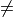None artificial damping will be applied to step 1

damping_factor2

IfNone artificial damping will be applied to step 2

timeInterval

float, the time interval where the outputs will be printed

stress_output

bool, tells to print stress outputs

force_output

bool, tells to print force outputs

output_requests

list, contains all the output variables that will be printed in the output

ncpus

Number of CPUs to run the jobs

Methods

 Attach the odb file into Abaqus Calculates the laminate stiffness matrix (ABD matrix) Calculates the predicted values for P1 and N1 based on empirically obtained formulae Calculates the KDF using the NASA SP-8007 guideline calc_partitions([thetadegs, pts]) Updates all circumferential and axial positions to partition create_model([force]) Triggers the routines to create the model in Abaqus Detach an odb file from Abaqus extract_fiber_orientation(ply_index, …) Get the fiber orientation at the centroid of each element extract_field_output([ignore]) Extract the current field output for a cylinder/cone from Abaqus Get a data grid representing the nodal offsets w.r.t. Get the thickness at the centroid of each element fr(z) Calculates the radius at a given z position from_DB([name_DB]) Fetch all the cone/cylinder data from the database get_step_name(step) Get the step name corresponding to an integer number plot_current_field_opened([ignore, plot_type]) Print the current field output for a cylinder/cone model from Abaqus plot_field_data(x, y, field[, …]) Print data field output to a file plot_msi_opened([plot_type]) Make an opened MSI (mid-surface imperfection) plot from the current conecyl model plot_orientation_opened(ply_index, use_elements) Make a fiber orientation plot from the current cone model plot_thickness_opened([plot_type]) Make an opened thickness plot from the current conecyl model Prepare the ConeCyl to be saved r_z_from_pt([pt]) Radius and the axial position from a given normalized position rebuild([force, save_rebuild]) Updates the properties of the current ConeCyl object transform_plot_data(thetas, zs, values, …) Transform coordinates of plot data, to prepare for plotting write_job([submit, wait, multiple_cores]) Writes the job of the corresponding Abaqus model
attach_results()[source]

Attach the odb file into Abaqus

If the odb file exists it will be attached in session.odbs, in Abaqus.

Note

Must be called from Abaqus

calc_ABD_matrix()[source]

Calculates the laminate stiffness matrix (ABD matrix)

Requires that all the laminate attributes are defines.

Returns
lamLaminate object.
calc_SPL_prediction()[source]

Calculates the predicted values for P1 and N1 based on empirically obtained formulae

Here P1 is the perturbation load (in N) at which a local snap-through (LST) appears at an axial load level equal to the global buckling load. N1 is the global buckling load that is obtained with P1 applied.

Returns
outtuple

2-tuple, containing the calculated values for P1 and N1

Notes

The empirical formulae (for now) do not take the full set of laminate properties (A, B, D) into account. Instead, the equivalent orthotropic material is calculated (based on the A-matrix only) and used in the formulae.

calc_nasaKDF()[source]

Calculates the KDF using the NASA SP-8007 guideline

Returns
nasaKDFfloat

The knock-down factor (KDF) calculates using the NASA SP-8007.

calc_partitions(thetadegs=None, pts=None)[source]

Updates all circumferential and axial positions to partition

This method reads all the imperfections and collects the circumferential positions thetadegs and the normalized meridional positions pts where partitions should be created. These two lists will be used in the routines to create an Abaqus model.

Parameters

Additional positions where circumferential partitions are desired

ptslist or None, optional

Additional positions where meridional partitions are desired

create_model(force=False)[source]

Triggers the routines to create the model in Abaqus

The auxiliary module _create_model.py is used, from where the functions _create_mesh(), _create_load_steps() and _create_loads_bcs() are executed in this order.

Note

Must be called from Abaqus

Note

When new functionalities have to be implemented or for any debugging purposes, one can conveniently change file _create_model.py directly, and using the __main__ section at the end of this file makes it easy to test whatever necessary methods. The tests can be repeatedly run doing:

import os

from desicos.abaqus.constants import DAHOME
os.chdir(os.path.join(DAHOME, 'conecyl'))
execfile('_create_model.py')

Parameters
forcebool, optional

Forces the model creation even if the finite element model corresponding to this ConeCyl object already exists.

detach_results(odb)[source]

Detach an odb file from Abaqus

Note

Must be called from Abaqus

Parameters
odbAbaqus’ Odb object.
extract_fiber_orientation(ply_index, use_elements)[source]

Get the fiber orientation at the centroid of each element

Parameters
ply_indexint

Index of the ply of interest

use_elementsbool

If True, use the actual element centroids (from Abaqus) If False, estimate their locations instead.

Returns
outtuple

Where out and out contain the circumferential (theta) and vertical (z) coordinates and out the corresponding values.

Notes

Must be called from Abaqus if use_elements == True

extract_field_output(ignore=[])[source]

Extract the current field output for a cylinder/cone from Abaqus

Parameters
ignorelist, optional

A list with the node ids to be ignored. It must contain any nodes outside the mapped mesh included in parts['part_name_shell'].nodes.

Returns
outtuple

Where out and out contain the circumferential (theta) and vertical (z) coordinates and out the corresponding values.

extract_msi_data()[source]

Get a data grid representing the nodal offsets w.r.t. the reference surface, caused by mid-surface imperfection(s).

Returns
outtuple

Where out and out contain the circumferential (theta) and vertical (z) coordinates and out the corresponding imperfection offsets.

Notes

Must be called from Abaqus

extract_thickness_data()[source]

Get the thickness at the centroid of each element

Returns
outtuple

Where out and out contain the circumferential (theta) and vertical (z) coordinates and out the corresponding thicknesses.

Notes

Must be called from Abaqus

fr(z)[source]

Calculates the radius at a given z position

Parameters
zfloat

Axial position from bottom to top.

Returns
rfloat

from_DB(name_DB='')[source]

Fetch all the cone/cylinder data from the database

Parameters
name_DBstr

Name of the corresponding desicos.conecylDB.ccs entry.

Returns
ccConeCyl object with the updated properties.
get_step_name(step)[source]

Get the step name corresponding to an integer number

Parameters
stepint

A step number. Abaqus’ “Initial” step does not count, such that step=1 will be the first step after the “Initial” step.

Returns
step_namestr

The step name.

plot_current_field_opened(ignore=[], plot_type=1, **kwargs)[source]

Print the current field output for a cylinder/cone model from Abaqus

Parameters
ignorelist, optional

A list with the node ids to be ignored. It must contain any nodes outside the mapped mesh included in parts['part_name_shell'].nodes.

plot_typeint, optional

For cylinders only 4 and 5 are valid. For cones all the following types can be used:

• 1: concave up (default for cones)

• 2: concave down

• 3: stretched closed

• 4: stretched opened (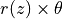vs.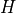)

• 5: stretched opened (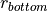vs.)

• 6: concave, starting at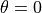kwargsdict

Other keyword args will be directly passed to plot_field_data See the documentation of that method for more details.

Returns
outtuple

Where out and out contain the circumferential and meridional grids of coordinates and out the corresponding field output.

plot_field_data(x, y, field, create_npz_only=False, ax=None, figsize=3.3, 3.3, save_png=True, aspect='equal', clean=True, outpath='', pngname='plot_from_abaqus.png', npzname='plot_from_abaqus.npz', pyname='plot_from_abaqus.py', num_levels=400, show_colorbar=True, lines=None)[source]

Print data field output to a file

Parameters
xnumpy.array

Grid of x-coordinates to plot

ynumpy.array

Grid of y-coordinates to plot

fieldnumpy.array

Grid of field data to plot

create_npz_onlybool, optional

If True only the data belonging to the desired field output will be saved in a .npz file, and no plotting is performed.

axAxesSubplot, optional

When ax is given, the contour plot will be created inside it.

figsizetuple, optional

The figure size given by (width, height).

save_pngbool, optional

Flag telling whether the contour should be saved to an image file.

aspectstr, optional

String that will be passed to the AxesSubplot.set_aspect() method.

cleanbool, optional

Clean axes ticks, grids, spines etc.

outpathstr, optional

Output path where the data from Abaqus and the plots are saved (see notes).

pngnamestr, optional

The file name for the generated image file.

npznamestr, optional

The file name for the generated npz file.

pynamestr, optional

The file name for the generated Python file.

num_levelsint, optional

Number of contour levels (higher values make the contour smoother).

show_colorbarbool, optional

Include a color bar in the figure.

lineslist, optional

List of lines to draw on top of the contour plot. Each line is either a 2-tuple (list of x-coords, list of y-coords), or a 2xN numpy array.

Notes

The data is saved using np.savez() into outpath as npzname with an accompanying script for plotting pyname, very handy when Matplotlib is not importable from Abaqus.

plot_msi_opened(plot_type=1, **kwargs)[source]

Make an opened MSI (mid-surface imperfection) plot from the current conecyl model

Parameters
plot_typeint, optional

For cylinders only 4 and 5 are valid. For cones all the following types can be used:

• 1: concave up (default for cones)

• 2: concave down

• 3: stretched closed

• 4: stretched opened (vs.)

• 5: stretched opened (vs.)

• 6: concave, starting atkwargsdict

Other keyword args will be passed to plot_field_data See the documentation of that method for more details.

Notes

Must be called from Abaqus

plot_orientation_opened(ply_index, use_elements, plot_type=1, **kwargs)[source]

Make a fiber orientation plot from the current cone model

Only valid for cones that have a ply piece imperfection.

Parameters
ply_indexint

Index of the ply of interest

use_elementsbool

If True, use the actual element centroids (from Abaqus) If False, estimate their locations instead.

plot_typeint, optional

For cones all the following types can be used:

• 1: concave up (default for cones)

• 2: concave down

• 3: stretched closed

• 4: stretched opened (vs.)

• 5: stretched opened (vs.)

• 6: concave, starting atkwargsdict

Other keyword args will be passed to plot_field_data See the documentation of that method for more details.

Notes

Must be called from Abaqus if use_elements == True

plot_thickness_opened(plot_type=1, **kwargs)[source]

Make an opened thickness plot from the current conecyl model

Parameters
plot_typeint, optional

For cylinders only 4 and 5 are valid. For cones all the following types can be used:

• 1: concave up (default for cones)

• 2: concave down

• 3: stretched closed

• 4: stretched opened (vs.)

• 5: stretched opened (vs.)

• 6: concave, starting atkwargsdict

Other keyword args will be passed to plot_field_data See the documentation of that method for more details.

Notes

Must be called from Abaqus

prepare_to_save()[source]

Prepare the ConeCyl to be saved

Any reference to Abaqus objects are removed in this method.

r_z_from_pt(pt=0.5)[source]

Radius and the axial position from a given normalized position

Parameters
ptfloat or np.ndarray

Normalized meridional position.

Returns
r, ztuple

The radius and the actual axial position at the given normalized position. It is a tuple of floats if pt is a float or a tuple of numpy.ndarray objects if pt is an array.

rebuild(force=False, save_rebuild=True)[source]

Updates the properties of the current ConeCyl object

Parameters
forcebool

Force the update even if it is already rebuilt (even if the rebuilt attribute is True).

save_rebuildbool

Tells if the rebuilt attribute should be True after the update.

transform_plot_data(thetas, zs, values, plot_type, wrap=True)[source]

Transform coordinates of plot data, to prepare for plotting

Parameters
thetasnumpy.array

Array of circumferential coordinates

zsnumpy.array

Array of vertical coordinates

valuesnumpy.array

Array of values

plot_typeint, optional

For cylinders only 4 and 5 are valid. For cones all the following types can be used:

• 1: concave up (default for cones)

• 2: concave down

• 3: stretched closed

• 4: stretched opened (vs.)

• 5: stretched opened (vs.)

• 6: concave, starting atwrapbool, optional

If True, wrap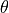-coordinates to within the correct range (either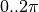or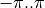).

Returns
outtuple

Where out and out contain the horizontal and vertical grids of coordinates and out the values.

write_job(submit=False, wait=True, multiple_cores=False)[source]

Writes the job of the corresponding Abaqus model

Note

Must be called from Abaqus

Parameters
submitbool, optional

If the job should be submitted.

waitbool, optional

If the routine should wait in case the job was submitted.

multiple_coresbool, optional

If multiple cores should be used in the run. Some licenses are limited to one core.

## Imperfections (desicos.abaqus.imperfections)¶

Embodies all imperfections that will be included in the finite element model.

The imperfections are grouped in one imperfection configuration ImpConf which knows how to add each imperfection type. In the example below a perturbation load and an axisymmetric imperfection are included. Note that the perturbation load is added in step 1 while the axial load in step 2, meaning that the perturbation load will kept a constant load while the axial load will be incremented along the non-linear analysis (see detailed description in ConeCyl):

from desicos.abaqus.conecyl import ConeCyl

cc = ConeCyl()
cc.from_DB('huehne_2008_z07')
cc.axial_step = 2
cc.create_model()

The finite element model is created in two steps:
• creating all the partitions at the moment the mesh is generated

• creating all the imperfections in a later step

Each imperfection has a method rebuild(), which must update two key properties thetadegs and pts, which are lists containing the necessary data for creating the partitions correctly.

Additionaly, each imperfection has a method create(), which creates the imperfection itself, looking for the right nodes that should be translated and so forth.

Invalid imperfections are identified when pt < 0. or pt > 1., which are just ignored and an error message is printed.

### Imperfection Configuration (desicos.abaqus.imperfections.impconf)¶

class desicos.abaqus.imperfections.impconf.ImpConf[source]

Imperfection Configuration

Created by default as one attribute of the ConeCyl object, accessed through:

cc = ConeCyl()
impconf = cc.impconf


If one has the impconf object and wants to access the corresponding ConeCyl object, the attribute conecyl can be used as examplified below. Note that if no ConeCyl is assigned to this imperfection configuration a None value will be obtained:

cc = impconf.conecyl


The imperfections are grouped in the attributes detailed below.

Attributes

Description

uneven_bottom_edge

uneven_top_edge

UnevenTopEdge object

list of PLoad (Perturbation Load) objects

dimples

list of Dimple (Dimple Imperfection) objects

axisymmetrics

list of Axisymmetric (Axisymmetric Imperfection) objects

lbmis

list of LBMI (Linear Buckling Mode-Shaped Imperfection) objects

tis

list of TI (Thickness Imperfection) objects

msis

list of MSI (Mid-Surface Imperfection) objects

cutouts

list of Cutout objects

ppi

PPI (Ply Piece Imperfection) object or None if not set

ffi

FFI (Fiber Fraction Imperfection) object or None if not set

Methods

 add_axisymmetric(pt, b, wb) Add an Axisymmetric Imperfection (AI) add_cb(thetadeg, pt, cbtotal[, step]) Add a Constant Amplitude Perturbation Buckle Imperfection add_cutout(thetadeg, pt, d[, …]) Add a cutout add_dimple(thetadeg, pt, a, b, wb) Add a Dimple Imperfection (DI) add_ffi(nominal_vf, E_matrix, nu_matrix, use_ti) Adds Fiber Fraction Imperfection (FFI) add_lbmi(mode, scaling_factor) Add a Linear Buckling Mode-shaped Imperfection (LBMI) add_measured_u3s_bottom_edge(thetadegs, u3s) Add a measured uneven bottom edge add_measured_u3s_top_edge(thetadegs, u3s) Add a measured uneven top edge add_msi([imp_ms, scaling_factor, …]) Add a Mid-Surface Imperfection (MSI) add_pload(thetadeg, pt, pltotal[, step]) Add a Perturbation Load add_ppi(info, extra_height) Adds Ply Piece Imperfection (PPI) add_shim_bottom_edge(thetadeg, thick, width) Add a Shim to the bottom edge add_shim_top_edge(thetadeg, thick, width) Add a Shim to the top edge add_ti(imp_thick, scaling_factor) Add Thickness Imperfection (TI)
 create rebuild
add_axisymmetric(pt, b, wb)[source]

Parameters
ptfloat

Normalized meridional position.

bfloat

Half-wave length.

wbfloat

Imperfection amplitude (amplitude of the half-wave).

Returns
axAxisymmetric object.
add_cb(thetadeg, pt, cbtotal, step=1)[source]

Add a Constant Amplitude Perturbation Buckle Imperfection

Parameters

Circumferential position.

ptfloat

Normalized meridional position.

cbtotalfloat

The magnitude of the constant buckle (it is always applied normally to the shell surface).

stepint

The step in which the constant buckle will be included. In step=1 the load is constant along the analysis while in step=2 the load is incremented.

Returns
cbCBamp object.
add_cutout(thetadeg, pt, d, drill_offset_deg=0.0, clearance_factor=0.75, numel_radial_edge=4, prop_around_cutout=None)[source]

Parameters

Circumferential position of the dimple.

ptfloat

Normalized meridional position.

dfloat

Diameter of the drilling machine.

drill_offset_degfloat, optional

Angular offset when the drilling is not normal to the shell surface. A positive offset means a positive rotation about theaxis, along the meridional plane.

clearance_factorfloat, optional

Fraction of the diameter to apply as clearance around the cutout. This clearance is partitoned and meshed separately from the rest of the cone / cylinder.

Number of elements along the radial edges about the cutout center. This parameter affects the aspect ratio of the elements inside the cutout area.

prop_around_cutoutdict, optional

Dictionary with keys:

• ‘mode’ : str (‘radius’ or ‘partition’)

• ‘stack’: list of floats

• ‘plyts’: list of floats

• ‘mat_names’: list of strings

.

Examples:

• Defining a property with 'mode'='radius':

prop_around_cutout = {
'stack': [0, 90, 0],
'plyts': [0.125, 0.125, 0.125],
'mat_names': ['Alum', 'Alum', 'Alum'],
}

• Defining a property with 'mode'='partition':

prop_around_cutout = {
'mode': 'partition',
'stack': [0, 90, 0],
'plyts': [0.125, 0.125, 0.125],
'mat_names': ['Alum', 'Alum', 'Alum'],
}


Note

mat_names must be a list of materials already created in the current model in Abaqus

Returns
cutoutCutout object.
add_dimple(thetadeg, pt, a, b, wb)[source]

Parameters

Circumferential position of the dimple.

afloat

Circumferential half-wave length of the dimple.

bfloat

Meridional half-wave length of the dimple.

wbfloat

Imperfection amplitude.

Returns
dDimple object.
add_ffi(nominal_vf, E_matrix, nu_matrix, use_ti, global_sf=None)[source]

There can be only one of these, so calling this function overrides the previous imperfection, if any.

Parameters
nominal_vffloat

Nominal fiber volume fraction of the material

E_matrixfloat

Young’s modulus of the matrix material

nu_matrixfloat

Poisson’s ratio of the matrix material

use_tibool

If True, create varying material properties according to the thickness imperfection data (if present).

global_sffloat or None

Global scaling factor to apply to the material thickness. Set to None to disable. The global scaling may be overridden by a thickness imperfection, if use_ti (see above) is True.

Returns
ffiFFI object.
add_lbmi(mode, scaling_factor)[source]

Add a Linear Buckling Mode-shaped Imperfection (LBMI)

Parameters
modeint

Mode number corresponding to this eigenvector.

scaling_factorfloat

Amplitude of this eigenvector when applied as an imperfection.

Returns
lbmiLBMI object.
add_measured_u3s_bottom_edge(thetadegs, u3s)[source]

Add a measured uneven bottom edge

Straightforward method to include measured data about the bottom edge imperfection.

Adopts the coordinate system of this figure when defining the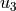displacements for eachvalue.

The edge imperfection that actually goes for each node is a linear interpolation of the measured values.

Parameters

The circumferential positions where the imperfect bottom edge was measured, in degrees.

u3slist

The measured imperfections representing displacements along theaxis of the adopted model.

add_measured_u3s_top_edge(thetadegs, u3s)[source]

Add a measured uneven top edge

Straightforward method to include measured data about the top edge imperfection.

Adopts the coordinate system of this figure when defining thedisplacements for eachvalue.

The edge imperfection that actually goes for each node is a linear interpolation of the measured values.

Parameters

The circumferential positions where the imperfect top edge was measured, in degrees.

u3slist

The measured imperfections representing displacements along theaxis of the adopted model.

add_msi(imp_ms='', scaling_factor=1.0, R_best_fit=None, H_measured=None, path=None, use_theta_z_format=True, rotatedeg=0.0, ignore_bot_h=True, ignore_top_h=True, stretch_H=False, c0=None, m0=None, n0=None, funcnum=None)[source]

Also called geometric imperfection.

If the imperfection is already included in the database only the corresponding entry imp_ms and the scaling factor need to be specified.

If the imperfection is not in the database one can specify the full path for the file containing the imperfection, the measured radius and height, as detailed below.

Parameters
imp_msstr, optional

Name of the imperfection in the database.

scaling_factorfloat, optional

Scaling factor applied to the original imperfection amplitude, usually to allow imperfection sensitivity studies.

R_best_fitfloat, optional

Best fit radius obtained with functions best_fit_cylinder() or best_fit_cone().

The semi-vertex angle of the measured sample (it is 0. for a cylinder).

H_measuredfloat, optional

The total height of the measured test specimen, including eventual resin rings at the edges.

pathstr, optional

Full path to the file containing the imperfection data.

use_theta_z_formatbool, optional

If the imperfection file is in the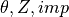format instead of the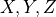format.

rotatedegfloat, optional

Rotation angle in degrees telling how much the imperfection pattern should be rotated about the(or) axis.

ignore_bot_hfloat, optional

Used to ignore nodes from the bottom resin ring. The default value True will use data from the bottom resin ring, if it exists.

ignore_top_hfloat, optional

Used to ignore nodes from the top resin ring. The default value True will use data from the top resin ring, if it exists.

stretch_Hbool, optional

If the measured imperfection does not cover the whole height it will be stretched. If stretch_H==True, ignore_bot_h and ignore_top_h are automatically set to False.

c0str or np.ndarray, optional

The coefficients representing the imperfection pattern. If supplied will overwrite the imperfection data passed using the other parameters. For more details see calc_c0().

m0int, optional

Number of terms along the meridian (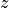) used to obtain c0, see calc_c0().

n0int, optional

Number of terms along the circumference () used to obtain c0, see calc_c0().

funcnumint, optional

The base function used to obtain c0, see calc_c0().

Returns
msiMSI object.
add_pload(thetadeg, pt, pltotal, step=1)[source]

Parameters

Circumferential position.

ptfloat

Normalized meridional position.

pltotalfloat

The magnitude of the perturbation load (it is always applied normally to the shell surface).

stepint

The step in which the perturbation load will be included. In step=1 the load is constant along the analysis while in step=2 the load is incremented.

Returns
ploadPLoad object.
add_ppi(info, extra_height)[source]

There can be only one of these, so calling this function overrides the previous imperfection, if any. Note: Applicable for cones only!

Parameters
infolist

List of dictionaries with info about the layup of this cone. See PPI for more details

extra_heightfloat

Extra height above and below the cone height (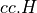) to consider in the ply placement model.

Returns
ppiPPI object.
add_shim_bottom_edge(thetadeg, thick, width)[source]

Add a Shim to the bottom edge

Parameters

Circumferential position where the shim starts.

thickfloat

Thickness of the shim.

widthfloat

Perimetrical width of the shim (along the shell perimeter).

Returns
shimShim object.
add_shim_top_edge(thetadeg, thick, width)[source]

Add a Shim to the top edge

Parameters

Circumferential position where the shim starts.

thickfloat

Thickness of the shim.

widthfloat

Perimetrical width of the shim (along the shell perimeter).

Returns
shimShim object.
add_ti(imp_thick, scaling_factor)[source]

The imperfection must be already included in the database (check this tutorial).

Parameters
imp_thickstr

Name of the thickness imperfection in the database.

scaling_factorfloat

Scaling factor applied to the original imperfection amplitude, usually to allow imperfection sensitivity studies.

Returns
tiTI object.

### Imperfection (desicos.abaqus.imperfections.imperfection)¶

class desicos.abaqus.imperfections.imperfection.Imperfection[source]

Base class for all imperfections

This class should be sub-classed when a new imperfection is created.

Methods

 create_sketch_plane get_xyz

### Axisymmetric (desicos.abaqus.imperfections.axisymmetric)¶

class desicos.abaqus.imperfections.axisymmetric.Axisymmetric(pt, b, wb)[source]

Axisymmetric Imperfection

The imperfection definition is a special case of the dimple imperfection proposed by Wullschleger and Meyer-Piening (2002) (see Dimple).

Methods

 Realizes the axisymmetric imperfection in the finite element model
 calc_amplitude create_sketch_plane get_xyz rebuild
create()[source]

Realizes the axisymmetric imperfection in the finite element model

The nodes corresponding to this imperfection are translated.

Note

Must be called from Abaqus.

### Dimple (desicos.abaqus.imperfections.dimple)¶

class desicos.abaqus.imperfections.dimple.Dimple(thetadeg, pt, a, b, wb)[source]

Dimple imperfection

References

Wullschleger, L. and Meyer-Piening, H.-R.. Buckling of geometrically imperfect cylindrical shells - definition of a buckling load. International Journal of Non-Linear Mechanics 37 (2002) 645-657.

Methods

 Realizes the dimple imperfection in the finite element model
 calc_amplitude create_sketch_plane get_xyz rebuild
create()[source]

Realizes the dimple imperfection in the finite element model

The nodes corresponding to this imperfection are translated.

Note

Must be called from Abaqus.

### LBMI (desicos.abaqus.imperfections.lbmi)¶

class desicos.abaqus.imperfections.lbmi.LBMI(mode, scaling_factor)[source]

Linear Buckling Mode-shaped Imperfection (LBMI)

Methods

 calc_amplitude create create_sketch_plane get_xyz rebuild

### Geometric Imperfection (desicos.abaqus.imperfections.msi)¶

class desicos.abaqus.imperfections.msi.MSI[source]

Mid-Surface Imperfection

The imperfections are applied using both an inverse-weighted interpolation algorithm, detailed in inv_weighted(), or a continuous fitting function, detailed in calc_c0().

The following attributes of the MSI object control the inverse-weighted algorithm:

Attribute

Description

ncp

int, number of closest points

power_parameter

float, power parameter

r_TOL

float, percentage tolerance to ignore noisy data, for example, when r_TOL=1. the points with a radius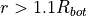Additional attributes are used to apply the imperfection into the finite element model when the inverse-weighted algorithm is selected.

Attribute

Description

imp_ms

str, an entry in the imperfection database, if an entry with this string key is found, it will overwrite the parameters: path, R_best_fit, H_measured

path

str, full path to the imperfection file

use_theta_z_format

bool, if the imperfection file is in theor in theformat

R_measured

float, best fit radius obtained with functions best_fit_cylinder() or best_fit_cone()

H_measured

float, height of the specimen for which the imperfection file corresponds to

scaling_factor

float, a scaling factor that is applied to the imperfection amplitude

sample_size

Avoids a memory overflow during runtime for large imperfection files

rotatedeg

float, rotation angle in degrees telling how much the imperfection pattern should be rotated about the(or) axis.

The following attributes of the MSI object control the continuous function-based algorithm:

Attribute

Description

c0

np.ndarray, coefficients giving the amplitude of each term in the approximation function given by funcnum. If specified overwrites even imp_ms

Note

The coefficients c0 must be calculated already considering rotatedeg using function calc_c0()

m0

int, number of terms along the meridional coordinate

n0

int, number of terms alog the circumferential coordinate

funcnum

int, the base function used for the approximation, as detailed in calc_c0()

scaling_factor

float, a scaling factor that is applied to the imperfection amplitude

Additional parameters that govern how the imperfection pattern will look like in the finite element model:

Attribute

Description

ignore_bot_h

Used to ignore nodes from the bottom resin ring. The default value True will automatically obtain the resin ring dimensions. Set to False or None if an imperfection pattern “extruded” to both edges is the desired behavior

ignore_top_h

Similar to ignore_bot_h, but for the top edge.

stretch_H

If the measured imperfection does not cover the whole height it will be stretched. If stretch_H is True, ignore_bot_h and ignore_top_h are automatically set to False

Methods

 Calculates the geometric imperfection of the finite element model create([force]) Applies the mid-surface imperfection in the finite element model
 print_to_file rebuild
calc_amplitude()[source]

Calculates the geometric imperfection of the finite element model

Note

Must be called from Abaqus.

Returns
max_ampfloat

The maximum absolute amplitude.

create(force=False)[source]

Applies the mid-surface imperfection in the finite element model

Note

Must be called from Abaqus.

Parameters
forcebool, optional

Creates the imperfection even when it is already created

desicos.abaqus.imperfections.msi.calc_msi_amplitude(cc, force=False)[source]

Calculates the mid-surface imperfection of a ConeCyl model

Note

Must be called from Abaqus.

Parameters
ccConeCyl object

The ConeCyl object already

forcebool, optional

Does not the check if the finite element model is already created.

Returns
max_ampfloat

The maximum absolute amplitude.

### Perturbation Load (desicos.abaqus.imperfections.pload)¶

class desicos.abaqus.imperfections.pload.PLoad(thetadeg, pt, pltotal, step=1)[source]

Methods

 Calculate the imperfection amplitude. Include the perturbation load.
 create_sketch_plane get_xyz rebuild
calc_amplitude()[source]

Calculate the imperfection amplitude.

The odb must be available and it will be used to extract the last frame of the first analysis step, corresponding to the constant loads.

create()[source]

The load step in which the perturbation load is included depends on the step parameter, which can be 1 or 2. If applied in the first step it will be kept constant, whereas in the second step it will be incremented.

The perturbation load is included after finding its corresponding vertice. The perturbation load is not created if its value is smaller then 0.1*TOL (see desicos.constants).

Note

Must be called from Abaqus.

### Thickness Imperfection (desicos.abaqus.imperfections.ti)¶

class desicos.abaqus.imperfections.ti.TI[source]

Thickness Imperfection

Assumes that a percentage variation of the laminate thickness can be represented by the same percentage veriation of each ply, i.e., each ply thickness is varied in order to reflect a given measured thickness imperfection field.

Methods

 Calculates the thickness imperfection amplitude create([force]) Creates the thickness imperfection
 rebuild
calc_amplitude()[source]

Calculates the thickness imperfection amplitude

Amplitude measured as the biggest difference between each layup thickness and the nominal thickness of the Cone/Cylinder, considering only the layups that are not suppressed.

Note

Must be called from Abaqus.

Returns
max_ampfloat

Maximum absolute imperfection amplitude.

create(force=False)[source]

Creates the thickness imperfection

The thickness imperfection is created assuming that each ply has the same contribution to the measured laminate thickness. Thus, a scaling factor is applied to the nominal thickness of each ply in order to macth the measured imperfection field.

Parameters
forcebool, optional

If True the thickness imperfection is applied even when it is already created.

### Uneven Edges (desicos.abaqus.imperfections.uneven_edges)¶

class desicos.abaqus.imperfections.uneven_edges.Shim(thetadeg, thick, width, edge=None)[source]

Represents a shim added to one of the edges

Attributes

Description

edge

An object of the class UnevenTopEdge

The circumferential position where the shim starts

thick

The shim thickness

width

The shim perimetrical width (along the shell perimeter)

class desicos.abaqus.imperfections.uneven_edges.UnevenBottomEdge(betadeg=None, omegadeg=None)[source]

Uneven Bottom Edge

The following attributes are taken into account: - misalignment of the bottom edge - presence of shims - measured uneven edge points

Attributes

Description

uneven_plate

bool: If the unevenness should be applied to the testing plate or to the test specimen

Misalignment of the bottom edge in degrees

Azimuth angle of the bottom edge misalignment in degrees.

shims

list of shims included to this edge

measured_u3s

Measured points describing the edge imperfection

Methods

 add_measured_u3s(thetadegs, u3s) Adds measured data to the uneven bottom edge add_shim(thetadeg, thick, width) Adds a shim to the uneven bottom edge Creates the uneven bottom edge imperfections
 calc_amplitude rebuild
add_measured_u3s(thetadegs, u3s)[source]

Adds measured data to the uneven bottom edge

The edge imperfection that actually goes for each node is a linear interpolation of the measured values.

Parameters

The circumferential positions where the imperfect bottom edge was measured, in degrees.

u3slist

The measured imperfections representing displacements along theaxis of the adopted model.

add_shim(thetadeg, thick, width)[source]

Adds a shim to the uneven bottom edge

Parameters

Circumferential position where the shim starts.

thickfloat

Thickness of the shim.

widthfloat

Perimetrical width of the shim (along the shell perimeter).

Returns
shimShim object.
create()[source]

Creates the uneven bottom edge imperfections

The uneven bottom edge will be represented by many GAP elements created in such a way to consider all the imperfections contained in the current UnevenBottomEdge object.

The output file cc.model_name + '_bottom_edge.gaps' will be created, where cc is the ConeCyl object that contains this UnevenBottomEdge object.

The following steps are executed:

• get thecoordinate of the bottom nodes from the shell and bottom resin rings

• get imperfection from the shims attribute

• get any additional imperfection of the bottom edge represented by measured_u3s

Assumptions:

• for a givencoordinate the uneven displacement is the same for all the shell and resin ring nodes

Note

Must be called from Abaqus

class desicos.abaqus.imperfections.uneven_edges.UnevenTopEdge(betadeg=None, omegadeg=None)[source]

Uneven Top Edge

The following attributes are taken into account: - misalignment of the top edge - presence of shims - measured uneven edge points

Attributes

Description

uneven_plate

bool: If the unevenness should be applied to the testing plate or to the test specimen

float: Misalignment of the top edge in degrees

float: Azimuth angle of the top edge misalignment in degrees

shims

list of shims included to this edge

measured_u3s

Measured points describing the edge imperfection

Methods

 add_measured_u3s(thetadegs, u3s) Adds measured data to the uneven top edge add_shim(thetadeg, thick, width) Adds a shim to the uneven top edge Creates the uneven top edge imperfections
 calc_amplitude rebuild
add_measured_u3s(thetadegs, u3s)[source]

Adds measured data to the uneven top edge

The edge imperfection that actually goes for each node is a linear interpolation of the measured values.

Parameters

The circumferential positions where the imperfect top edge was measured, in degrees.

u3slist

The measured imperfections representing displacements along theaxis of the adopted model.

add_shim(thetadeg, thick, width)[source]

Adds a shim to the uneven top edge

Parameters

Circumferential position where the shim starts.

thickfloat

Thickness of the shim.

widthfloat

Perimetrical width of the shim (along the shell perimeter).

Returns
shimShim object.
create()[source]

Creates the uneven top edge imperfections

The uneven top edge will be represented by many GAP elements created in such a way to consider all the imperfections contained in the current UnevenTopEdge object.

The output file cc.model_name + '_top_edge.gaps' will be created, where cc is the ConeCyl object that contains this UnevenTopEdge object.

The following steps are executed:

• get thecoordinate of the top nodes from the shell and top resin rings

• get imperfection from the shims attribute

• get any additional imperfection of the top edge represented by measured_u3s

• include effect of the misalignment angle betadeg

Assumptions:

• for a givencoordinate the uneven displacement is the same for all the shell and resin ring nodes, but the load asymmetry angle self.betadeg may change this equality. The contribution due to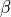is given by: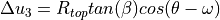Note

Must be called from Abaqus

### Cutout (desicos.abaqus.imperfections.cutout)¶

class desicos.abaqus.imperfections.cutout.Cutout(thetadeg, pt, d, drill_offset_deg=0.0, clearance_factor=0.75, numel_radial_edge=4, prop_around_cutout=None)[source]
Parameters

Circumferential position of the dimple.

ptfloat

Normalized meridional position.

dfloat

Diameter of the drilling machine.

drill_offset_degfloat, optional

Angular offset when the drilling is not normal to the shell surface. A positive offset means a positive rotation about theaxis, along the meridional plane.

clearance_factorfloat, optional

Fraction of the diameter to apply as clearance around the cutout. This clearance is partitoned and meshed separately from the rest of the cone / cylinder.

Number of elements along the radial edges about the cutout center. This parameter affects the aspect ratio of the elements inside the cutout area.

prop_around_cutoutdict, optional

Dictionary with keys:

• ‘mode’ : str (‘radius’ or ‘partition’)

• ‘stack’: list of floats

• ‘plyts’: list of floats

• ‘mat_names’: list of strings

.

Examples:

• Defining a property with 'mode'='radius':

prop_around_cutout = {
'stack': [0, 90, 0],
'plyts': [0.125, 0.125, 0.125],
'mat_names': ['Alum', 'Alum', 'Alum'],
}

• Defining a property with 'mode'='partition':

prop_around_cutout = {
'mode': 'partition',
'stack': [0, 90, 0],
'plyts': [0.125, 0.125, 0.125],
'mat_names': ['Alum', 'Alum', 'Alum'],
}


Note

mat_names must be a list of materials already created in the current model in Abaqus

Methods

 calc_amplitude create create_prop_around_cutout rebuild

### Ply Piece Imperfection (desicos.abaqus.imperfections.ppi)¶

class desicos.abaqus.imperfections.ppi.PPI(info, extra_height=0)[source]

Ply Piece Imperfection

Laminating a cone with a finite number of ply pieces causes deviations between the nominal fiber angle (e.g. 30 degrees) and the actual angle, which varies with the location on the cone. This imperfection can be used to include that effect in the simulation.

Attributes

Description

info

list with info about the layup of this cone. Length of the list should be at least equal to the number of plies. Each entry is a dict, containing:

• starting_position: float, Radius in the flattened cone ((s, phi)-coordinate system) where the origin line (L0) of the basic ply piece intersects the horizontal axis

• max_width: float, maximum width of a single ply piece.

• rel_ang_offset: float, optional, default is 0. Relative angular offset (0..1) to be used when positioning the pieces in this ply. Used to avoid overlapping of seams, when multiple plies have the same orientation.

• eccentricity: float, eccentricity param (range 0…1) that controls the positioning of the ply piece relative to the origin line. Optional, the default value is dependent on the nominal fiber angle:

1. 0.5 if cc.stack[i] == 0

2. 0.0 if cc.stack[i] > 0

3. 1.0 if cc.stack[i] < 0

extra_height

float, extra height above and below the cone height () to consider in the ply placement model.

Notes

This imperfection only works for cones, not for cylinders.

Methods

 Actually create the imperfection fiber_orientation(ply_index, coords) Determine the local fiber orientation at a set of coordinates, given in the global Cartesian (x, y, z) coordinate system. gcs_to_unfolded(x, y, z) Convert global xyz coordinates to the unfolded (eta, zeta)-csys. get_ply_lines(ply_index[, center_theta_zero]) Obtain a series of lines that can be used to draw all ply pieces. unfolded_to_gcs(eta, zeta[, approx_phi, …]) Convert unfolded (eta, zeta)-coordinates to the global coordinate system.
 calc_amplitude create_sketch_plane get_xyz rebuild
create()[source]

Actually create the imperfection

This modifies all composite layups to replace their existing (constant) ply orientations with values that are defined by a discrete field.

Note

Must be called from Abaqus.

fiber_orientation(ply_index, coords)[source]

Determine the local fiber orientation at a set of coordinates, given in the global Cartesian (x, y, z) coordinate system. If points are not covered by any ply piece, NaN is returned for those

Parameters
ply_indexint

Index of the ply of interest

coordsnumpy.array

Two-dimensional array containing one row per point and the x-, y- and z-coordinates of each point as columns.

Returns
thetasnumpy.array

Local fiber angle at each given point in the ply, in degrees.

gcs_to_unfolded(x, y, z)[source]

Convert global xyz coordinates to the unfolded (eta, zeta)-csys.

Parameters
xfloat or numpy array

X-coordinates in global Cartesian coordinate system

yfloat or numpy array

Y-coordinates in global Cartesian coordinate system

zfloat or numpy array

Z-coordinates in global Cartesian coordinate system

Returns
outtuple

A 2-tuple, where out contains the eta-coordinate(s) and out the zeta-coordinate(s) corresponding to the given point(s).

Notes

Input coordinates should be on the surface of the cone.

get_ply_lines(ply_index, center_theta_zero=True)[source]

Obtain a series of lines that can be used to draw all ply pieces.

Parameters
ply_indexint

Index of ply to construct lines for.

center_theta_zerobool

Plot the ply pieces in the -pi…pi range, instead of 0..2pi

Returns
lineslist

List of lines. Each line is a 2-tuple (thetas, zs), containing a list of circumferential coordinates and a list of vertical coordinates of the points along the line.

unfolded_to_gcs(eta, zeta, approx_phi=0.0, cylindrical=False)[source]

Convert unfolded (eta, zeta)-coordinates to the global coordinate system.

Parameters
etafloat or numpy array

Horizontal coordinates in the unfolded coordinate system.

zetafloat or numpy array

Vertical coordinates in the unfolded coordinate system.

approx_phifloat, optional

As an intermediate step, the (eta, zeta)-coordinates are converted to polar (s, phi)-coordinates. This transformation is multivalued, as (s, phi + 2pi) and such is also a valid result. Resolve this ambiguity by choosing the value of phi closest to approx_phi, so within the (approx_phi - pi, approx_phi + pi) range.

cylindricalbool, optional

Whether to return output values in a Cartesian (if False) or cylindrical (if True) coordinate system. Default is False.

Returns
outtuple

A 3-tuple, containing (depending on the parameter cylindrical) either (x, y, z) or (r, theta, z)-coordinates.

### Fiber Fraction Imperfection (desicos.abaqus.imperfections.ffi)¶

class desicos.abaqus.imperfections.ffi.FFI(nominal_vf, E_matrix, nu_matrix, use_ti, global_sf=None)[source]

Fiber Fraction Imperfection

Thickness variations are generally caused by a varying amount of matrix, while the amount of fibers remains constant. Thus, the actual fiber volume fraction is higher in thinner sections of the material. This imperfection aims to include that effect in the model, by adjusting the material properties.

Attributes

Description

nominal_vf

float, nominal fiber volume fraction

E_matrix

float, Young’s modulus of the matrix material

nu_matrix

float, Poisson’s ratio of the matrix material

use_ti

bool, if True, create varying material properties according to the thickness imperfection data (if present).

global_sf

float or None, global scaling factor to apply to the material thickness. Set to None to disable. The global scaling may be overridden by a thickness imperfection, if use_ti (see above) is True.

created

bool, True after the imperfection has been created.

Attributes
scaling_factor

Methods

 Calculates the imperfection amplitude calc_scaled_laminaprop(laminaprop, …) Calculate material properties of a lamina with a scaled thickness. Actually create the imperfection Call this function after the thickness imperfection(s) are applied, to modify the material properties as well, if needed.
 create_sketch_plane get_xyz rebuild
calc_amplitude()[source]

Calculates the imperfection amplitude

Amplitude measured as the biggest thickness difference between the actual and nominal layup thickness of the Cone/Cylinder, considering only the layups that have this imperfection applied.

Note

Must be called from Abaqus.

Returns
max_ampfloat

Maximum absolute imperfection amplitude.

calc_scaled_laminaprop(laminaprop, scaling_factor)[source]

Calculate material properties of a lamina with a scaled thickness.

Calculates the new lamina properties, if a given material (lamina) is scaled in thickness by a given factor. The new material properties are calculated from the properties of the fiber and the matrix, using the composition rule (for E11 and nu12) and the corrected composition rule (for E22, G12, G13 and G23).

The matrix properties are to be supplied by the user. The fiber properties are calculated based on the original (nominal) lamina properties, using the inverse of the respective composition rule.

Parameters
laminaproptuple

Material properties (E11, E12, nu12, G12, G13, G23) of the lamina at the nominal thickness

scaling_factorfloat

Scaling factor that is to be applied to the ply thickness. The total amount of fibers is assumed to remain constant, thus the actual fiber volume fraction is inversely proportional to this scaling factor.

Returns
new_laminaproptuple

Lamina properties of the lamina with a scaled thickness

create()[source]

Actually create the imperfection

Note

Must be called from Abaqus.

update_after_tis()[source]

Call this function after the thickness imperfection(s) are applied, to modify the material properties as well, if needed.

## Apply Imperfections (desicos.abaqus.apply_imperfections)¶

Routines to apply geometric and thickness imperfections into the finite element model.

desicos.abaqus.apply_imperfections.calc_translations_ABAQUS(imperfection_file_name, model_name, part_name, H_model, H_measured, R_model, R_best_fit=None, semi_angle=0.0, stretch_H=False, z_offset_bot=None, rotatedeg=0.0, scaling_factor=1.0, r_TOL=1.0, num_closest_points=5, power_parameter=2, use_theta_z_format=True, ignore_bot_h=None, ignore_top_h=None, sample_size=None, T=None)[source]

Reads an imperfection file and calculates the nodal translations

Parameters
imperfection_file_namestr

Full path to the imperfection file.

model_namestr

Model name.

part_namestr

Part name.

H_modelfloat

Total height of the model where the imperfections will be applied to, considering also eventual resin rings.

H_measuredfloat

The total height of the measured test specimen, including eventual resin rings at the edges.

R_modelfloat

Radius at the bottom edge of the model where the imperfections will be applied to.

R_best_fitfloat, optional

Best fit radius obtained with functions best_fit_cylinder() or best_fit_cone().

semi_anglefloat, optional

Cone semi-vertex angle in degrees, when applicable.

stretch_Hbool, optional

If the measured imperfection data should be stretched to the current model (which may happen when H_model!=H_measured.

z_offset_botfloat, optional

It is common to have the measured data not covering the whole test specimen, and therefore it will be centralized, if a non-centralized position is desired this parameter can be used for the adjustment.

rotatedegfloat, optional

Rotation angle in degrees telling how much the imperfection pattern should be rotated about the(or) axis.

scaling_factorfloat, optional

A scaling factor that can be used to study the imperfection sensitivity.

r_TOLfloat, optional

Percentage tolerance to ignore noisy data from the measurements.

num_closest_pointsint, optional
power_parameterfloat, optional
use_theta_z_formatbool, optional

If the new formatshould be used instead of the old.

ignore_bot_hNone or float, optional

Used to ignore nodes from the bottom resin ring.

ignore_top_hNone or float, optional

Used to ignore nodes from the top resin ring.

sample_sizeint, optional

If the input file containing the measured data is too large it may be required to limit the sample size.

TNone or np.ndarray, optional

A transformation matrix (cf. transf_matrix()) required when the mesh is not in the default coordinate system.

desicos.abaqus.apply_imperfections.change_thickness_ABAQUS(imperfection_file_name, model_name, part_name, stack, t_model, t_measured, H_model, H_measured, R_model, R_best_fit=None, number_of_sets=None, semi_angle=0.0, stretch_H=False, z_offset_bot=None, scaling_factor=1.0, num_closest_points=5, power_parameter=2, elems_t=None, t_set=None, use_theta_z_format=False)[source]

Applies a given thickness imperfection to the finite element model

Assumes that a percentage variation of the laminate thickness can be represented by the same percentage veriation of each ply, i.e., each ply thickness is varied in order to reflect a given measured thickness imperfection field.

Parameters
imperfection_file_namestr

Full path to the imperfection file.

model_namestr

Model name.

part_namestr

Part name.

stacklist

The stacking sequence of the current model with each angle given in degrees.

t_modelfloat

The nominal shell thickness of the current model.

t_measuredfloat

The nominal thickness of the measured specimen.

H_modelfloat

Total height of the model where the imperfections will be applied to, considering also eventual resin rings.

H_measuredfloat

The total height of the measured test specimen, including eventual resin rings at the edges.

R_modelfloat

Radius at the bottom edge of the model where the imperfections will be applied to.

R_best_fitfloat, optional

Best fit radius obtained with functions best_fit_cylinder() or best_fit_cone().

number_of_setsint, optional

Defines in how many levels the thicknesses should be divided. If None it will be based on the input file, and if the threshold of 100 is exceeded, 10 sections are used.

semi_anglefloat, optional

Cone semi-vertex angle in degrees, when applicable.

stretch_Hbool, optional

If the measured imperfection data should be stretched to the current model (which may happen when H_model!=H_measured.

z_offset_botfloat, optional

It is common to have the measured data not covering the whole test specimen, and therefore it will be centralized, if a non-centralized position is desired this parameter can be used for the adjustment.

scaling_factorfloat, optional

A scaling factor that can be used to study the imperfection sensitivity.

num_closest_pointsint, optional
power_parameterfloat, optional
elems_tnp.ndarray, optional

Interpolated thickness for each element. Can be used to avoid the same interpolation to be performed twice.

t_setset, optional

A set object containing the unique thicknesses that will be used to create the new properties.

use_theta_z_formatbool, optional

If the new formatshould be used instead of the old.

desicos.abaqus.apply_imperfections.translate_nodes_ABAQUS(imperfection_file_name, model_name, part_name, H_model, H_measured, R_model, R_best_fit=None, semi_angle=0.0, stretch_H=False, z_offset_bot=None, rotatedeg=0.0, scaling_factor=1.0, r_TOL=1.0, num_closest_points=5, power_parameter=2, nodal_translations=None, use_theta_z_format=False, ignore_bot_h=None, ignore_top_h=None, sample_size=None, T=None)[source]

Translates the nodes in Abaqus based on imperfection data

The imperfection amplitude for each node is calculated using an inversed weight function (see desicos.conecylDB.interpolate.inv_weighted()).

Parameters
imperfection_file_namestr

The full path to the imperfection file, which must be a file with three columns containing the x, y, z coordinates when use_theta_z_format=False or containing x, theta, amplitude when use_theta_z_format=True.

model_namestr

Must be a valid key in the dictionary mdb.models, in the interactive Python inside Abaqus.

part_namestr

Must be a valid key in the dictionary mdb.models[model_name].parts, in the interactive Python inside Abaqus.

H_modelfloat

Total height of the model where the imperfections will be applied to, considering also eventual resin rings.

H_measuredfloat

The total height of the measured test specimen, including eventual resin rings at the edges.

R_modelfloat

The radius of the current model. In case of cones this should be the bottom radius.

R_best_fitfloat, optional

Best fit radius obtained with functions best_fit_cylinder() or best_fit_cone().

semi_anglefloat, optional

The cone semi-vertex angle (a null value indicates that a cylinder is beeing analyzed).

stretch_Hfloat, optional

A boolean indicating if the imperfection pattern should be stretched when applied to the model. The measurement systems usually cannot obtain data for the whole surface, making it an option to stretch the data to fit the whole surface. In case stretch_H=False the measured data of the extremities will be extruded up to the end of the domain.

z_offset_botfloat, optional

This parameter allows the analyst to adjust the height of the measured data about the model, when the measured data is not available for the whole domain.

rotatedegfloat, optional

Rotation angle in degrees telling how much the imperfection pattern should be rotated about the(or) axis.

scaling_factorfloat, optional

The scaling factor that will multiply the calculated imperfection amplitude.

r_TOLfloat, optional

Parameter to ignore noisy data in the imperfection file, the points with a radius higher than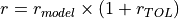will not be considered in the interpolation.

num_closest_pointsint, optional
power_parameterint, optional
nodal_translationsNone or numpy.ndarray, optional

An array containing the interpolated traslations, which is passed to avoid repeated calls to the interpolation functions.

use_theta_z_formatbool, optional

A boolean to indicate whether the imperfection file contains x, y, z positions or theta, z, amplitude.

ignore_bot_hNone or float, optional

Used to ignore nodes from the bottom resin ring.

ignore_top_hNone or float, optional

Used to ignore nodes from the top resin ring.

sample_sizeint, optional

If the input file containing the measured data is too large it may be required to limit the sample size.

TNone or np.ndarray, optional

A transformation matrix (cf. transf_matrix()) required when the mesh is not in the default coordinate system.

Returns
nodal_translationsnumpy.ndarray

A 2-D array containing the translations x, y, z for each column.

Notes

Despite the nodal traslations are returned all the nodes belonging to this model will already be translated.

desicos.abaqus.apply_imperfections.translate_nodes_ABAQUS_c0(m0, n0, c0, funcnum, model_name, part_name, H_model, semi_angle=0.0, scaling_factor=1.0, fem_meridian_bot2top=True, ignore_bot_h=None, ignore_top_h=None, T=None)[source]

Translates the nodes in Abaqus based on a Fourier series

The Fourier Series can be a half-sine, half-cosine or a complete Fourier Series as detailed in desicos.conecylDB.fit_data.calc_c0().

Parameters
m0int

Number of terms along the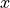coordinate.

n0int

Number of terms along thecoordinate.

c0numpy.ndarray

The coefficients that will give the imperfection pattern.

funcnumint

The function type, as detailed in desicos.conecylDB.fit_data.calc_c0().

model_namestr

Must be a valid key in the dictionary mdb.models, in the interactive Python inside Abaqus.

part_namestr

Must be a valid key in the dictionary mdb.models[model_name].parts, in the interactive Python inside Abaqus.

H_modelfloat

Total height of the model where the imperfections will be applied to, considering also eventual resin rings.

semi_anglefloat, optional

The cone semi-vertex angle (a null value indicates that a cylinder is beeing analyzed).

scaling_factorfloat, optional

The scaling factor that will multiply c0 when applying the imperfections.

fem_meridian_bot2topbool, optional

A boolean indicating if the finite element has theaxis starting at the bottom or at the top.

ignore_bot_hNone or float, optional

Used to ignore nodes from the bottom resin ring.

ignore_top_hNone or float, optional

Used to ignore nodes from the top resin ring.

TNone or np.ndarray, optional

A transformation matrix (cf. transf_matrix()) required when the mesh is not in the default coordinate system.

Returns
nodal_translationsnumpy.ndarray

A 2-D array containing the translations x, y, z for each column.

Notes

Despite the nodal traslations are returned all the nodes belonging to this model will be already translated.

## Abaqus Study (desicos.abaqus.study)¶

class desicos.abaqus.study.Study[source]

Study grouping many ConeCyl objects.

The objective of this class is to save any study where different models are included in one .cae file. THe

Methods

 Applies all geometric imperfections in this study Applies all thickness imperfections in this study Creates the run file which can be called from any Python save([path]) Save the current study
apply_msis()[source]

Applies all geometric imperfections in this study

It assumes the same MSI for all the ConeCyl objects that are in the ccs container.

apply_tis()[source]

Applies all thickness imperfections in this study

It assumes the same TI for all the ConeCyl objects that are in the ccs container.

create_run_file()[source]

Creates the run file which can be called from any Python

The file is stored in the self.study_dir folder.

save(path='')[source]

Save the current study

Parameters
pathstr, optional

The study is saved into the self.tmp_dir folder if path is not given.

## Utilities (desicos.abaqus.utils)¶

Includes all utilities functions that can be executed without Abaqus.

desicos.abaqus.utils.utils.add2list(lst, value, tol=1e-09)[source]

Adds a value to a list if it doesn’t exist within a given tolerance

Performs more or less like the Python build-in set(), but with a tolerance associated.

Parameters
lstlist

The input list.

valuefloat

The value to be compared with each element of the input list.

Returns
outlist

Extended input list.

desicos.abaqus.utils.utils.empirical_P1_isotropic(r, t, E, nu)[source]

taken from Wang et al. (2008). An empirical formula for the critical perturbation load

desicos.abaqus.utils.utils.get_book_sheet(excel_name, sheet_name)[source]

Gets an Excel Worksheet from a given file name

Parameters
excel_namestr

The full path for the desired Excel file.

sheet_namestr

The name of the desired Excel Worksheet.

Returns
workbook, sheettuple

A tuple with an xlwt.Workbook and an xlwt.Worksheet object.

desicos.abaqus.utils.utils.index_within_linspace(a, value)[source]

Returns the index where the value fits better

Parameters
anp.ndarray or list

The values where the best index will be found.

valuefloat

The value to be compared with each value in a.

Returns
iint

The index where value fits better in a.

desicos.abaqus.utils.utils.make_uniform_cells(x1, x2, values)[source]

Transform a grid to have uniform cells when plotted.

This is done by replacing each data point in the grid (assumed to be the element centroid) by four points, having the same value. These points are placed such that they would be near the element corners, so the element interior will have a uniform color in a contour plot.

Parameters
x1numpy.array

2D array containing the first coordinate (in whatever csys).

x2numpy.array

2D array containing the second coordinate (in whatever csys).

valuesnumpy.array

2D array containing the data values.

Notes

Input coordinates x1 and x2 are assumed to be regularly spaced. The type of coordinates (Cartesian, cylindrical, …) does not matter.

### Geometries (desicos.abaqus.utils.geom)¶

class desicos.abaqus.utils.geom.Plane[source]

Plane object

Defined by the attributes:

Attribute

Description

thetadeg

float, circumferential position in degrees

p1

tuple, a tuple containing the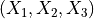coordinates for point 1

p2

tuple, a tuple containing thecoordinates for point 2

p3

tuple, a tuple containing thecoordinates for point 3

part

Abaqus Part object corresponding to this plane

feature

Abaqus Part Feature object corresponding to this plane

datum

Abaqus Part Datum object corresponding to this plane

Methods

 Creates the plane based on three points
create()[source]

Creates the plane based on three points

The three points must be previously stored in the attributes p1, p2 and p3.

## Utilities Abaqus (desicos.abaqus.abaqus_functions)¶

Includes all utilities functions that must be executed from Abaqus.

desicos.abaqus.abaqus_functions.configure_session()[source]

Improve layout and colors of the current figures in visualization

desicos.abaqus.abaqus_functions.create_composite_layup(name, stack, plyts, mat_names, region, part, part_csys, symmetric=False, scaling_factor=1.0, axis_normal=2)[source]

Creates a composite layup

Parameters
namestr

Name of the new composite layup.

stacklist

Stacking sequence represented by a list of orientations in degress. The stacking sequence starts inwards a ends outwards. The 0 degree angle is along the axial direction and the angles are measured using the right-hand rule with the normal direction being normal to the shell surface pointing outwards.

plytslist

List containing the ply thicknesses.

mat_nameslist

List containing the material name for each ply.

regionan Abaqus Region object

The region consisting of geometric faces, where this laminate will be assigned to.

partan Abaqus part Object

A part object where the layup will be created.

part_csysa valid Datum object

The cylindrical coordinate system of the part object.

symmetricbool, optional

A boolean telling whether the laminate is symmetric.

scaling_factorfloat, optional

A scaling factor to be applied to each ply thickness. Used to apply thickness imperfection in some cases.

axis_normalint, optional

Reference

desicos.abaqus.abaqus_functions.create_isotropic_section(name, mat_names, region, part, model, T, Sect_name, OFFTS)[source]

Creates an isotropic section

desicos.abaqus.abaqus_functions.create_sketch_plane(cc, entity)[source]

Creates a sketch plane tangent to the shell surface

Parameters
ccConeCyl object
entityobject

Any object with the attribute: thetadeg, usually a Imperfection.

Returns
planePlane object
desicos.abaqus.abaqus_functions.edit_keywords(mod, text, before_pattern=None, insert=False)[source]

Edit the keywords to add commands not available in Abaqus CAE

Parameters
modAbaqus Model object

The model for which the keywords will be edited.

textstr

The text to be included.

before_patternstr, optional

One pattern used to find where to put the given text.

insertbool, optional

Insert the text instead of replacing it.

desicos.abaqus.abaqus_functions.modify_composite_layup(part, layup_name, modify_func)[source]

Modify plies within a composite layup

Directly modififying plies within a CompositeLayup is not possible, as the plies are read-only after creation. This function emulates modifying, by deleting and then re-creating plies, with modifications.

Parameters
partan Abaqus part object

The part that the to-be-modified layup is attached to.

layup_namestr

Name of the layup that is to be modified.

modify_funcfunction

Function that will be called for each ply. It should take as arguments the ply index and a dictionary of keyword arguments. This dictionary contains all keyword arguments that would re-create the original ply, if passed to the CompositePly-constructor. This function should should make the necessary changes this dictionary and then return it. The returned dictionary will then be used to create the new ply.

desicos.abaqus.abaqus_functions.print_png(filename)[source]

Print a png file from the current viewport

Parameters
filenamestr

The name of the output png file.

desicos.abaqus.abaqus_functions.set_default_view(cc)[source]

Set a default view in order to compare figures from different models

Parameters
ccConeCyl object

## Stringers (desicos.abaqus.stringers)¶

All stringer classes are grouped in this module.

Each stringer has a method create(), that will be called from desicos.abaqus.conecyl.create_model(). Below there is an example about how to add the the blade type of stringers in a ConeCyl model:

import numpy as np

from desicos.abaqus.conecyl import ConeCyl

cc = ConeCyl()

cc.from_DB('huehne_2008_z07')

stack = [0, 0, 90, 90, -45, +45]
laminaprop = (142.5e3, 8.7e3, 0.28, 5.1e3, 5.1e3, 5.1e3)
laminaprops = [laminaprop for _ in stack]
plyts = [0.125 for _ in stack]

for thetadeg in np.linspace(0, 360, 12, endpoint=False):
stack=stack, plyts=plyts, laminaprops=laminaprops, numel_flange=5)
cc.create_model()


### Stringers Configuration (desicos.abaqus.stringers.stringerconf)¶

class desicos.abaqus.stringers.stringerconf.StringerConf[source]

Stringer configuration class

Methods

 add_blade_composite(thetadeg, wbot, wtop, …) Add a composite blade stringer add_blade_isotropic(thetadeg, wbot, wtop, h, …) Add an isotropic blade stringer
 create
add_blade_composite(thetadeg, wbot, wtop, stack, plyts, laminaprops, numel_flange=4)[source]

Parameters

Circumferential position in degrees.

wbotfloat

Flange width at the bottom edge.

wtopfloat

Flange width at the top edge.

stacklist

Laminate stacking sequence.

plytslist

Ply thicknesses.

laminapropslist

The properties for each lamina.

numel_flangeint, optional

The number of elements along the width.

add_blade_isotropic(thetadeg, wbot, wtop, h, E, nu, numel_flange=4)[source]

Implemented as a special case of the composite stringer for isotropic material.

Parameters

Circumferential position in degrees.

wbotfloat

Flange width at the bottom edge.

wtopfloat

Flange width at the top edge.

hfloat

Stringer thickness

Efloat

Young Modulus

nufloat

Poisson’s ratio

numel_flangeint, optional

The number of elements along the width.

### Blade Stringers (desicos.abaqus.stringers.blade)¶

class desicos.abaqus.stringers.blade.BladeComposite(thetadeg, wbot, wtop, stack, plyts, laminaprops, numel_flange=4)[source]

Parameters

Circumferential position in degrees.

wbotfloat

Flange width at the bottom edge.

wtopfloat

Flange width at the top edge.

stacklist

Laminate stacking sequence.

plytslist

Ply thicknesses.

laminapropslist

The properties for each lamina.

numel_flangeint, optional

The number of elements along the width.

Attributes

Methods

 create
class desicos.abaqus.stringers.blade.BladeIsotropic(thetadeg, wbot, wtop, h, E, nu, numel_flange=4)[source]

Parameters

Circumferential position in degrees.

wbotfloat

Flange width at the bottom edge.

wtopfloat

Flange width at the top edge.

hfloat

Stringer thickness

Efloat

Young Modulus

nufloat

Poisson’s ratio

numel_flangeint, optional

The number of elements along the width.

Attributes